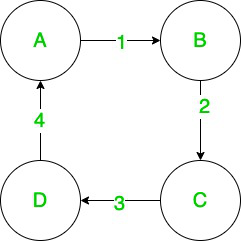# How to Represent Graph Using Incidence Matrix in Java?

• Last Updated : 11 Dec, 2020

An incidence matrix is simply a matrix that contains information about the relationship between objects (or instances) of any two classes. The rows of the matrix represent one class of objects while the columns represent the other class. Any coordinate (x, y) in the matrix represents the relationship between the xth Element of the first class and the yth element of the second class.

For example, let’s say that an incidence matrix A represents the relation between cars and manufacturers. If we let the rows represent the cars and the columns represent the manufacturers, any coordinate (x, y) in the matrix would give us information about whether the xth car was manufactured by the yth manufacturer.

Graphs and Incidence Matrices

An incidence matrix is a matrix (say A) of size n x m where n is the number of vertices, and m is the number of edges in the graph. Any element Ai,j in the matrix represents information about the relation between vertex i and edge j. The value could be different depending on the type of graph and relations being represented.

Let’s take a look at representing an undirected graph as an incidence matrix. The input representation will be an adjacency matrix, but you can adapt this to any other representation with simple logic.

#### Obtaining an Incidence Matrix from an Adjacency Matrix with Java

The most common graph representation you would encounter in most places is that of an adjacency matrix. Thus, here we show how to obtain an incidence matrix from an adjacency matrix, where Ai,j represents the edge number connecting vertices i and j.

The approach we’ll follow to convert adjacency matrix to incidence matrix is:

1. Count the number of edges in the graph by counting the number of non-zero values in the adjacency matrix. For undirected graphs, this can be done in O(n(n-1)/2).
2. Create an incidence matrix of size vertices x edges where each column would represent the incidence of an edge on all the rows crossing that column.
3. Similarly to the walk-in step 1., we walk through the adjacency matrix again to find out the vertices connected by the various edges. For each edge found in the adjacency matrix, we mark its incident vertices as 1 in the incidence matrix.

As an example, let’s represent the following graph as in the incidence matrix:A simple graph with 4 vertices and 4 edges

## Java

 `// Java program to convert adjacency matrix``// to incidence matrix`` ` `import` `java.io.*;`` ` `class` `GFG {``    ``public` `int``[][] adjacencyMatToIncidenceMat(``int``[][] adj)``    ``{``        ``int` `vertices = adj.length, edges = ``0``;`` ` `        ``// count number of edges in the graph``        ``for` `(``int` `i = ``0``; i < adj.length; i++) {``            ``for` `(``int` `j = i + ``1``; j < adj[i].length; j++) {``                ``if` `(adj[i][j] > ``0``)``                    ``edges++;``            ``}``        ``}`` ` `        ``// construct incidence matrix``        ``int``[][] incidenceMat = ``new` `int``[adj.length][edges];``        ``for` `(``int` `i = ``0``; i < adj.length; i++) {``           ` `            ``for` `(``int` `j = i + ``1``; j < adj[i].length; j++) {``                ``int` `edgeNumber = adj[i][j];`` ` `                ``if` `(edgeNumber > ``0``) {``                    ``incidenceMat[i][edgeNumber - ``1``] = ``1``;``                    ``incidenceMat[j][edgeNumber - ``1``] = ``1``;``                ``}``            ``}``           ` `        ``}`` ` `        ``return` `incidenceMat;``    ``}`` ` `    ``// driver code``    ``public` `static` `void` `main(String[] args)``    ``{``        ``GFG gfg = ``new` `GFG();``        ``int``[][] adj = {``            ``{ ``0``, ``1``, ``0``, ``4` `},``            ``{ ``1``, ``0``, ``2``, ``0` `},``            ``{ ``0``, ``2``, ``0``, ``3` `},``            ``{ ``4``, ``0``, ``3``, ``0` `},``        ``};``        ``int``[][] incidence = gfg.adjacencyMatToIncidenceMat(adj);`` ` `        ``for` `(``int``[] row : incidence) {``            ``for` `(``int` `val : row) {``                ``System.out.print(val);``            ``}``            ``System.out.println();``        ``}``    ``}``}`

Output

```1001
1100
0110
0011```

Time Complexity: O(n(n-1)) where n is the number of vertices.

My Personal Notes arrow_drop_up